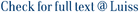We study long-term Markov decision processes (MDPs) and gambling houses, with applications to any partial observation MDPs with finitely many states and zero-sum repeated games with an informed controller. We consider a decision maker who is maximizing the weighted sum ∑t ≥ 1 trt, where rt is the expected reward of the t-th stage. We prove the existence of a very strong notion of long-term value called general uniform value, representing the fact that the decision maker can play well independently of the evaluations (t)t ≥ 1 over stages, provided the total variation (or impatience) ∑t≥1∣∣θt+1−θt∣∣ is small enough. This result generalizes previous results of the literature that focus on arithmetic means and discounted evaluations. Moreover, we give a variational characterization of the general uniform value via the introduction of appropriate invariant measures for the decision problems, generalizing the fundamental theorem of gambling or the Aumann–Maschler cav(u) formula for repeated games with incomplete information. Apart the introduction of appropriate invariant measures, the main innovation in our proofs is the introduction of a new metric, d*, such that partial observation MDPs and repeated games with an informed controller may be associated with auxiliary problems that are nonexpansive with respect to d*. Given two Borel probabilities over a compact subset X of a normed vector space, we define d∗(u,v)=supf∈D1∣∣u(f)−v(f)∣∣, where D1 is the set of functions satisfying ∀ x, y ∈ X, ∀ a, b ≥ 0, af(x) − bf(y) ≤ ‖ax − by‖. The particular case where X is a simplex endowed with the L1-norm is particularly interesting: d* is the largest distance over the probabilities with finite support over X, which makes every disintegration nonexpansive. Moreover, we obtain a Kantorovich–Rubinstein-type duality formula for d*(u, v), involving couples of measures (α, β) over X × X such that the first marginal of α is u and the second marginal of β is v.

Long-Term Values in Markov Decision Processes and Repeated Games, and a New Distance for Probability Spaces / Renault, J; Venel, X. - In: MATHEMATICS OF OPERATIONS RESEARCH. - ISSN 0364-765X. - 42:2(2017), pp. 349-376. [10.1287/moor.2016.0814]

### Long-Term Values in Markov Decision Processes and Repeated Games, and a New Distance for Probability Spaces

#### Abstract

We study long-term Markov decision processes (MDPs) and gambling houses, with applications to any partial observation MDPs with finitely many states and zero-sum repeated games with an informed controller. We consider a decision maker who is maximizing the weighted sum ∑t ≥ 1 trt, where rt is the expected reward of the t-th stage. We prove the existence of a very strong notion of long-term value called general uniform value, representing the fact that the decision maker can play well independently of the evaluations (t)t ≥ 1 over stages, provided the total variation (or impatience) ∑t≥1∣∣θt+1−θt∣∣ is small enough. This result generalizes previous results of the literature that focus on arithmetic means and discounted evaluations. Moreover, we give a variational characterization of the general uniform value via the introduction of appropriate invariant measures for the decision problems, generalizing the fundamental theorem of gambling or the Aumann–Maschler cav(u) formula for repeated games with incomplete information. Apart the introduction of appropriate invariant measures, the main innovation in our proofs is the introduction of a new metric, d*, such that partial observation MDPs and repeated games with an informed controller may be associated with auxiliary problems that are nonexpansive with respect to d*. Given two Borel probabilities over a compact subset X of a normed vector space, we define d∗(u,v)=supf∈D1∣∣u(f)−v(f)∣∣, where D1 is the set of functions satisfying ∀ x, y ∈ X, ∀ a, b ≥ 0, af(x) − bf(y) ≤ ‖ax − by‖. The particular case where X is a simplex endowed with the L1-norm is particularly interesting: d* is the largest distance over the probabilities with finite support over X, which makes every disintegration nonexpansive. Moreover, we obtain a Kantorovich–Rubinstein-type duality formula for d*(u, v), involving couples of measures (α, β) over X × X such that the first marginal of α is u and the second marginal of β is v.
##### Scheda breve Scheda completa Scheda completa (DC)gambling houses, partial observation Markov decision processes, repeated games, Wasserstein metric, uniform value, characterization of the value
Long-Term Values in Markov Decision Processes and Repeated Games, and a New Distance for Probability Spaces / Renault, J; Venel, X. - In: MATHEMATICS OF OPERATIONS RESEARCH. - ISSN 0364-765X. - 42:2(2017), pp. 349-376. [10.1287/moor.2016.0814]
File in questo prodotto:
File
Renault_Venel_2017.pdf

Open Access

Tipologia: Documento in Pre-print
Licenza: DRM non definito
Dimensione 572.47 kB
Long-Term Values in Markov Decision Processes and Repeated Games and a New Distance for Probability Spaces.pdf

Solo gestori archivio

Tipologia: Versione dell'editore
Licenza: DRM non definito
Dimensione 425.26 kB
Utilizza questo identificativo per citare o creare un link a questo documento: `http://hdl.handle.net/11385/197395`
•9
•9